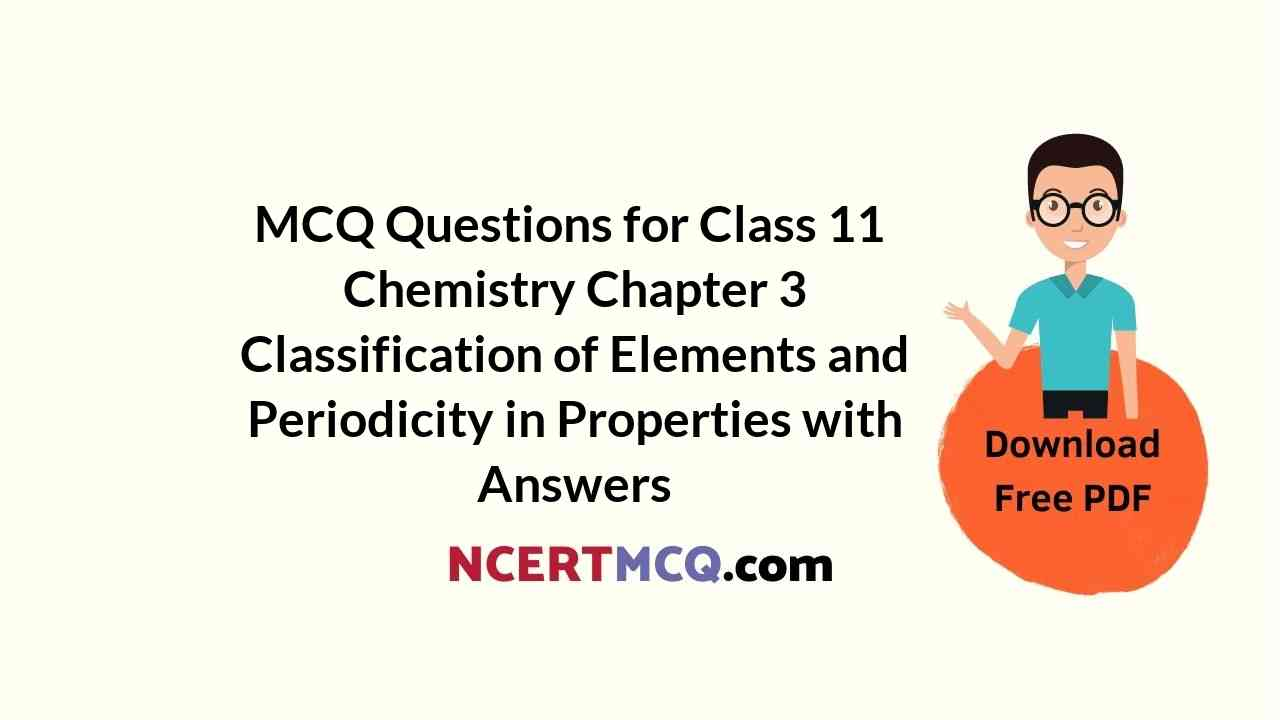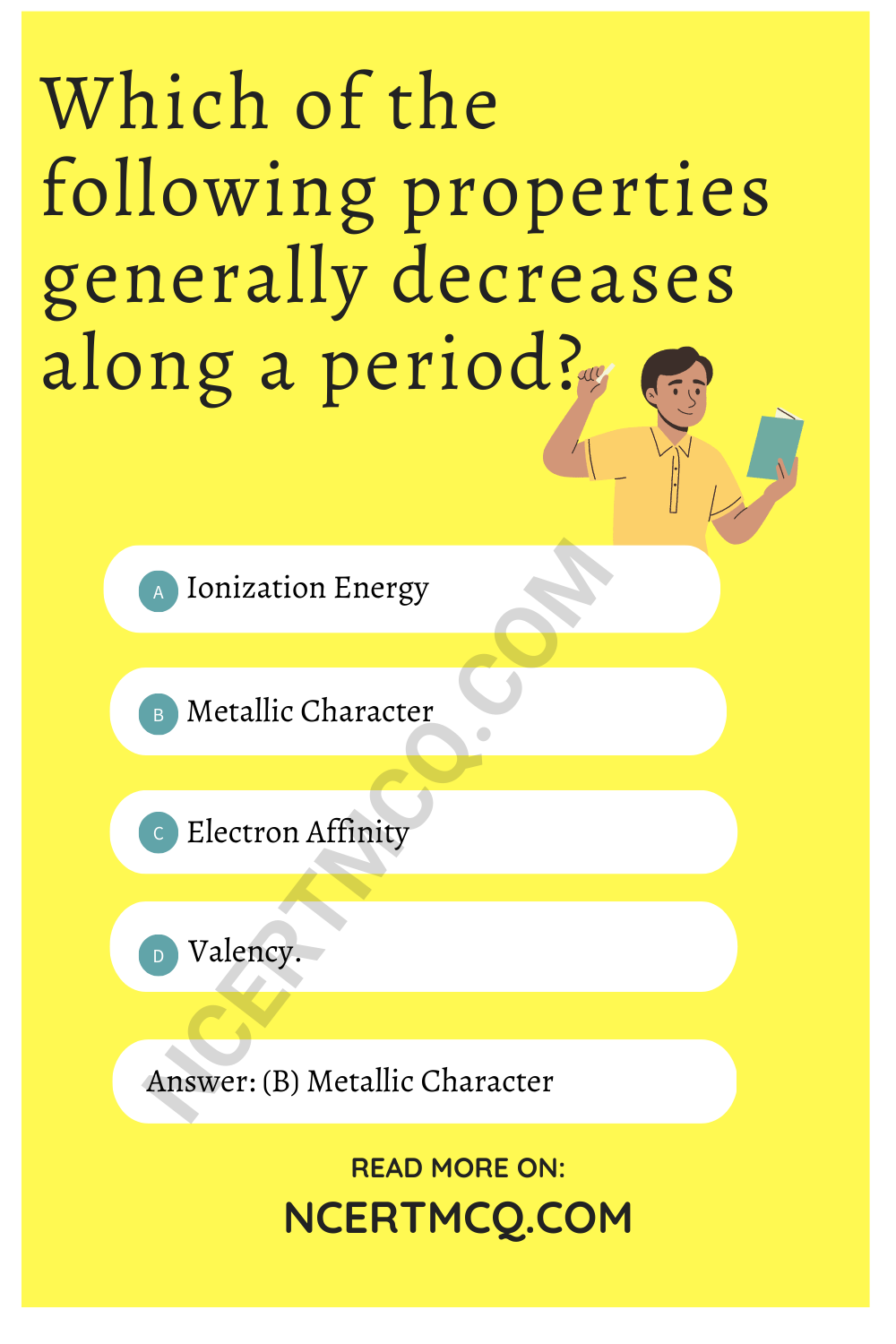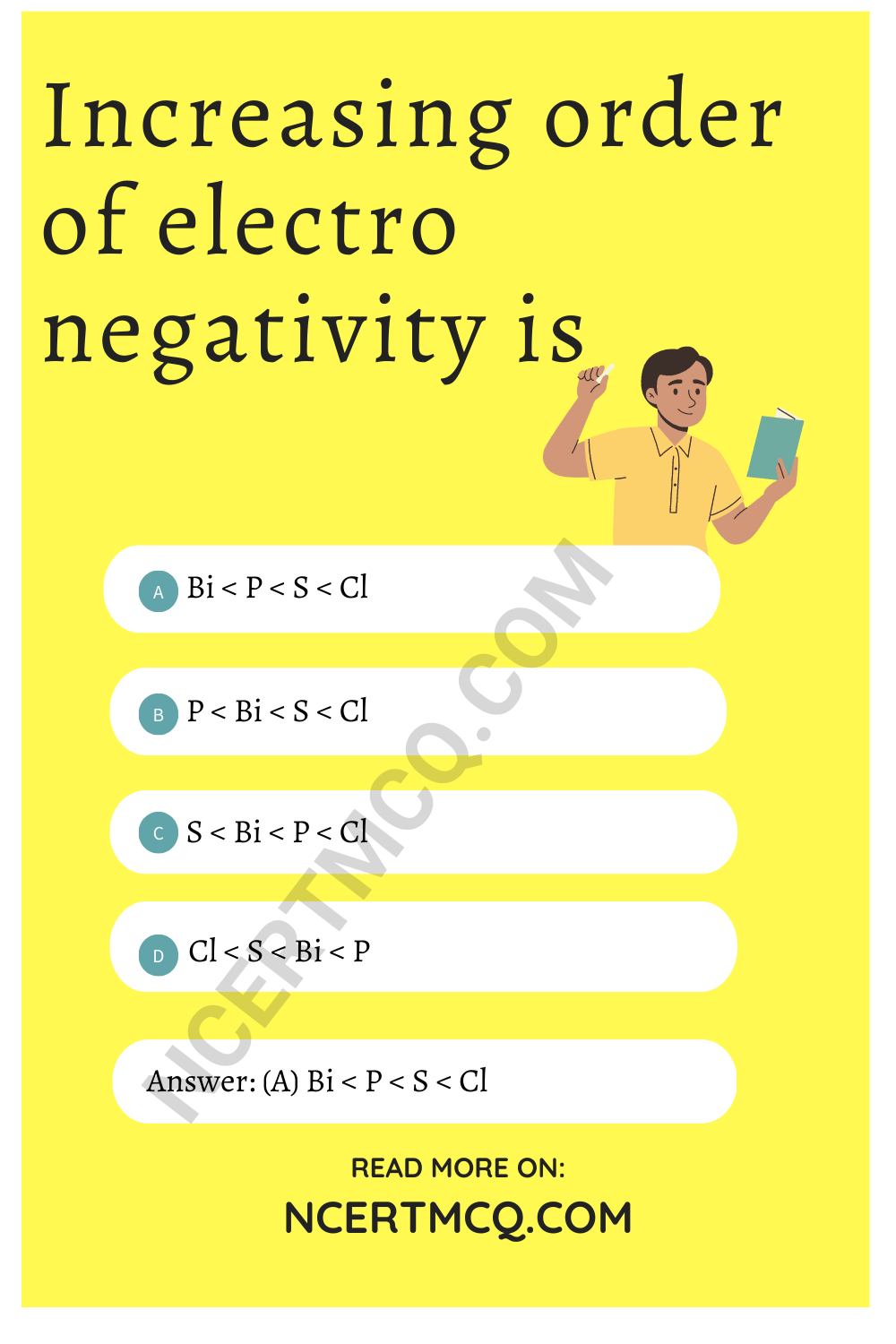Check the below NCERT MCQ Questions for Class 11 Chemistry Chapter 3 Classification of Elements and Periodicity in Properties with Answers Pdf free download. MCQ Questions for Class 11 Chemistry with Answers were prepared based on the latest exam pattern. We have provided Classification of Elements and Periodicity in Properties Class 11 Chemistry MCQs Questions with Answers to help students understand the concept very well.

## Classification of Elements and Periodicity in Properties Class 11 MCQs Questions with Answers

Classification Of Elements And Periodicity In Properties MCQ Question 1.
The chemistry of lithium is very similar to that of magnesium even though they are placed in different groups. Its reason is:
(a) Both are found together in nature
(b) Both have nearly the same size
(c) Both have similar electronic configuration
(d) The ratio of their charge and size (i.e. charge density) is nearly the same

Answer: (d) The ratio of their charge and size (i.e. charge density) is nearly the same
Explanation:
The chemistry of lithium is very similar to that of magnesium even though they are placed in different groups because of diagonal relationship.

Class 11 Chemistry Chapter 3 MCQ Question 2.
The element with atomic number 35 belongs to
(a) d – Block
(b) f – Block
(c) p – Block
(d) s – Block

Explanation:
The electronic configuration of element with atomic number 35 is [Ar]3d104s2 4p5. The valence electron belongs to p block. Therefore, it is a p-block element.

MCQ On Periodic Classification Of Elements Class 11 Pdf With Answers Question 3.
The correct order of first ionization potential among following elements, Be, B, C, N and O is
(a) B < Be < C < O < N
(b) B < Be < C < N < O
(c) Be < B < C < N < O
(d) Be < B < C < O < N

Answer: (a) B < Be < C < O < N
Explanation:
The energy required to remove the most loosely bound electron from an isolated gaseous atom is called the ionisation energy. The ionisation potential decreases as the size of the atom decreases. Atoms with fully or partly filled orbitals have high ionisation potential.

Class 11 Chemistry Chapter 3 MCQ With Answers Question 4.
Representative elements are those which belong to
(a) p and d – Block
(b) s and d – Block
(c) s and p – Block
(d) s and f – Block

Answer: (c) s and p – Block
Explanation:
Elements in which all the inner shells are complete but outer shell is incomplete is known as representative elements i.e. Those elements which have less than 8 electrons in outermost shell are representative.
s and p block elements except inert gas is known as representative elements.

Classification Of Elements And Periodicity In Properties Class 11 MCQ Question 5.
Which of the following properties generally decreases along a period?
(a) Ionization Energy
(b) Metallic Character
(c) Electron Affinity
(d) Valency.

Explanation:
The IE, EA increases along the period. The valency initially increases then decreases. The metallic character decreases along the period.MCQ Questions For Class 11 Chemistry Chapter 3 Question 6.
On the Paulings electronegativity scale the element next to F is
(a) N
(b) Cl
(c) O
(d) Ne.

Explanation:
Pauling explained electronegativity as the power of an atom in a molecule to attract electrons towards it. When we analyse the trend in periodic table, we can see that the degree of electronegativity decreases while going down the groups, while it increases across the periods. In the case of a covalent bond, based on the bond energies, Pauling calculated the differences in electronegativity between atoms in the bond and assigned a value of 4 to fluorine, which is the most electronegative element, and others were calculated with respect to that value. Hence, on paulings scale, the element next to fluorine is Oxygen.

Periodic Classification Of Elements Class 11 MCQ Question 7.
The group number, number of valence electrons, and valency of an element with the atomic number 15, respectively, are:
(a) 16, 5 and 2
(b) 15, 5 and 3
(c) 16, 6 and 3
(d) 15, 6 and 2

Answer: (b) 15, 5 and 3
Explanation:
Atomic number (Z) =15 =P → [Ne] 3s² 3p³
Phosphorus belongs to 15th group
Number of valence electrons 3s²3p³ = 5 and valency = 3 in ground state.

Ch 3 Chemistry Class 11 MCQ Question 8.
Which of the following oxides is amphoteric in character?
(a) SnO2
(b) CO2
(c) SiO2
(d) CaO

Explanation:
CaO is basic; CO2 is acidic; SiO2 is weakly acidic. SnO2 is amphoteric.

Chapter 3 Chemistry Class 11 MCQ Question 9.
In the modern periodic table, the period indicates the value of:
(a) Atomic Number
(b) Atomic Mass
(c) Principal Quantum Number
(d) Azimuthal Quantum Number

Explanation:
The periodic table is a tabular arrangement of the chemical elements, organized on the basis of their atomic number (number of protons in the nucleus), electron configurations, and recurring chemical properties.
The value of the principal quantum number (n) for the outermost shell or the valence shell indicates a period in the Modern periodic table.

Chemistry Class 11 Chapter 3 MCQ Question 10.
In the long form of the periodic table, the valence shell electronic configuration of 5s²5p4 corresponds to the element present in:
(a) Group 16 and period 6
(b) Group 17 and period 6
(c) Group 16 and period 5
(d) Group 17 and period 5

Answer: (c) Group 16 and period 5
Explanation:
Tellurium (Te) has 5s²5p4 valence shell configuration. It belongs to group 16 and present in period 5 of the periodic table.

Class 11 Chemistry Ch 3 MCQ Question 11.
Arrange S, O and Se in ascending order of electron affinity
(a) Se < S < O
(b) Se < O < S
(c) S < O < Se
(d) S < Se < O

Answer: (a) Se < S < O
Explanation:
Correct order of electron affinity is Se < S < O. In a group electron affinity decreases with increase in atomic number.

Periodic Classification Of Elements Class 11 MCQ With Answers Question 12.
In the modern periodic table , the period indicates the value of:
(a) Atomic Number
(b) Atomic Mass
(c) Principal Quantum Number
(d) Azimuthal Quantum Number

Explanation:
The periodic table is a tabular arrangement of the chemical elements, organized on the basis of their atomic number (number of protons in the nucleus), electron configurations, and recurring chemical properties.
The value of the principal quantum number (n) for the outermost shell or the valence shell indicates a period in the Modern periodic table.

MCQ Questions On Periodic Classification Of Elements Class 11 Question 13.
The electronic configuration of an element is 1s², 2s² 2p6, 3s² 3p³. What is the atomic number of the element which is just below the above element in the periodic table
(a) 31
(b) 34
(c) 33
(d) 49

Explanation:
33−1s²2s²2p63s²3p63d104s²4p³

MCQ Of Chapter 3 Chemistry Class 11 Question 14.
The reduction in atomic size with increase in atomic number is a characteristic of elements of-
(a) d−block
(b) f−block
(d) High atomic masses

Explanation:
The reduction in atomic size with increase in atomic number is a characteristic of elements of f- block. It is known as lanthanoid contraction and actinoid contraction. This is due to poor shielding of electrons present in f subshell.

MCQ On Classification Of Elements And Periodicity In Properties Question 15.
The number of elements in the 5th period of the periodic table is
(a) 3
(b) 9
(c) 8
(d) 18

Explanation:
While filling 5th shell according to Aufbau principle 5s, 5p, 4d filled so 2 + 6 + 10 = 18 electrons or elements are present in 5th shell. Further we start filling the 4d orbital which can take 10 electrons. So in the 4th principal quantum energy states, we can fill 18 electrons. Thus 5th period has 18 elements.

Class 11 Chemistry Chapter 3 MCQ Questions With Answers Question 16.
The electronic configuration of halogen is
(a) ns² np6
(b) ns² np3
(c) ns² np5
(d) ns²

Explanation:
Halogens has 7 electrons in his valance shell (ns²np5).

MCQ Of Ch 3 Chemistry Class 11 Question 17.
Which of the following forms the most stable gaseous negative ion?
(a) F
(b) Cl
(c) Br
(d) I

Explanation:
The element which forms the most stable gaseous negative ion is fluorine.

Classification Of Elements And Periodicity In Properties MCQ Pdf Question 18.
On the Paulings electro negativity scale the element next to F is
(a) N
(b) Cl
(c) O
(d) Ne.

Explanation:
Pauling explained electro negativity as the power of an atom in a molecule to attract electrons towards it. When we analyse the trend in periodic table, we can see that the degree of electro negativity decreases while going down the groups, while it increases across the periods. In the case of a covalent bond, based on the bond energies, Pauling calculated the differences in electro negativity between atoms in the bond and assigned a value of 4 to fluorine, which is the most electro negative element, and others were calculated with respect to that value. Hence, on paulings scale, the element next to fluorine is Oxygen.

Chapter 3 Class 11 Chemistry MCQ Question 19.
The element californium belongs to a family of :
(a) Alkali metal family
(b) Actinide series
(c) Alkaline earth family
(d) Lanthanide series

Explanation:
Atomic number of californium is 98 and its electronic configuration is
Rn86 7s² 5f10
so it is a f-block element and as it is in 7th period, it is a part of actinide series.

MCQ Of Classification Of Elements And Periodicity In Properties Question 20.
Increasing order of electro negativity is
(a) Bi < P < S < Cl
(b) P < Bi < S < Cl
(c) S < Bi < P < Cl
(d) Cl < S < Bi < P